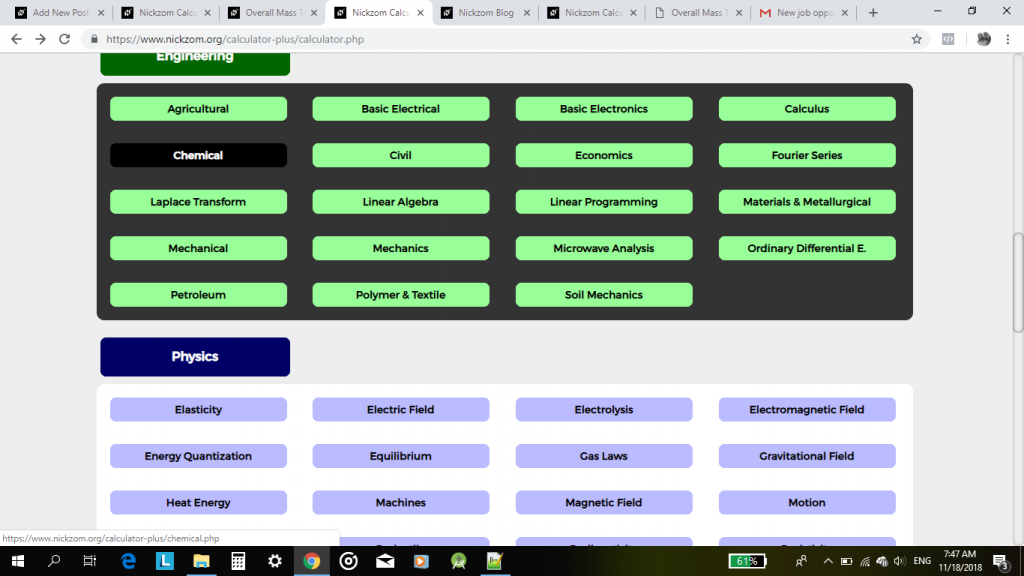## The Calculator Encyclopedia Calculates the Overall Mass Transfer Co-efficient in Crystallization for Chemical Engineering

Nickzom Calculator+ calculates virtually all the mathematical problems faced by an undergraduate of chemical engineering.

Today, we would be handling the obtaining of answers on overall mass transfer co-efficient which is under crystallization in Chemical engineering by Nickzom Calculator+.

One can access Nickzom Calculator+ (Professional Version) – The Calculator Encyclopedia via any of these channels:

First and foremost, if I want to get answers to my crystallization problem on Overall Mass Transfer Coefficient, I will proceed to my calculator map and click on Chemical (under Engineering Section).